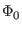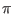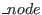Next: .disto Line Up: .dc Line Previous: .dc Line   Contents   Index

### Phase-Mode DC Analysis

The Josephson junction device has unique behavior which complicates simulation with a SPICE-type simulator (see 2.17.1.1). Central is the idea of phase, which is a quantum-mechanical concept and is generally invisible in the non-quantum world. However with superconductivity, and with Josephson junctions in particular, phase becomes not only observable, but a critical parameter describing these devices and the circuits that contain them.

A general superconducting circuit contains loops of inductors and Josephson junctions. When quiescent, the voltage at all nodes of such a circuit is identically zero, and thus it would seem to be impossible to use a SPICE-type simulator to perform DC analysis on this type of circuit. However, by using phase, which is nonzero at each node, instead of voltage, one can perform DC analysis, using phase-mode DC''.

WRspice after release 4.3.3 offers a DC analysis capability which uses phase-mode for circuits containing Josephson junctions. Unlike strictly phase-mode simulators, WRspice allows a mixture of phase (inductors and Josephson junctions) and voltage mode components.

Within WRspice, every node connected to a Josephson junction, inductor, or lossless transmission line (treated as an inductor in DC analysis) has a Phase'' flag set. This indicates that the computed value is phase, not voltage, for these nodes. We have to special-case the matrix loading functions for inductors, mutual inductors, lossless transmission lines, and resistors. Other devices are treated normally.

A Josephson junction can be modeled by the basic formula

I = Icsin(V(i, j))

where V(i, j) is the voltage'' difference between nodes i and j (across the junction) which is actually the phase. Inductors look like resistors:

I =V(i, j)/2L

Where V(i, j) is the phase difference across the inductor. Mutual inductance adds similar cross terms.

Capacitors are completely ignored as in normal DC analysis. The treatment of resistors is slightly complicated. The connected nodes can be Ground'', Phase'', or Voltage'' type. If both nodes are Voltage or Ground, the resistor is loaded normally. If both nodes are Phase or Ground, the resistor is not loaded at all. The interresting case is when one node is Phase, the other Voltage. In this case, we load the resistor as if the phase node is actually ground (node number 0). In addition we load a voltage-controlled current source template that injects current into the phase node of value V(voltage)/Resistance .

Resistors are the bridge between normal voltage-mode devices and phase nodes. Some circuits may require introduction of resistors to get correct results. For example, assume a Josephson junction logic gate driving a CMOS comparator circuit. If the input MOS gate is connected directly to the junction, the DC operating point will be incorrect, as the comparator will see phase as input. However, if a resistor separates the MOS gate from the junction, the comparator input will be zero, as it should be.

There is (at present) a topological requirement that all phase nodes must be at ground potential. This means that for a network of Josephson junctions and inductors, there must be a ground connection to one of these devices. A nonzero voltage source connected to an inductor, which is connected to a resistor to ground, although a perfectly valid circuit, will fail. One must use the equivalent consisting of the voltage source connected to a resistor, connected to the inductor which is grounded. This satisfies the two topological requirements:

Rule 1
There must be a resistor between a voltage-mode device and a phase-mode device, no direct connections allowed.

Rule 2
Every phase-node subnet must have a connection to ground, so all phase nodes are at ground potential.

With this bit of information, and the warning that controlled sources can cause unexpected behavior, the DC analysis using thes technique can apply to general circuits containing Josephson junctions.

Of course, for this to work, no Josephson junction can be biased above its critical current or nonconvergence results. Both DC operating point and DC sweep are available, as is AC analysis. Noise analysis is available with the internal Josephson junction model. This new hybrid technique appears to be an important advance, which should avoid the long-standing need to use uic'' and ramp sources up from zero, and makes available DC sweep analysis, and for the first time in any simulator AC small-signal analysis.Next: .disto Line Up: .dc Line Previous: .dc Line   Contents   Index
Stephen R. Whiteley 2022-09-18